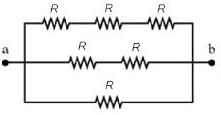# What is the equivalent resistance between points a and b in the figure if R=120Ω. R ab=?What is the equivalent resistance between points a and b in the figure if R=120Ω.
Rab=